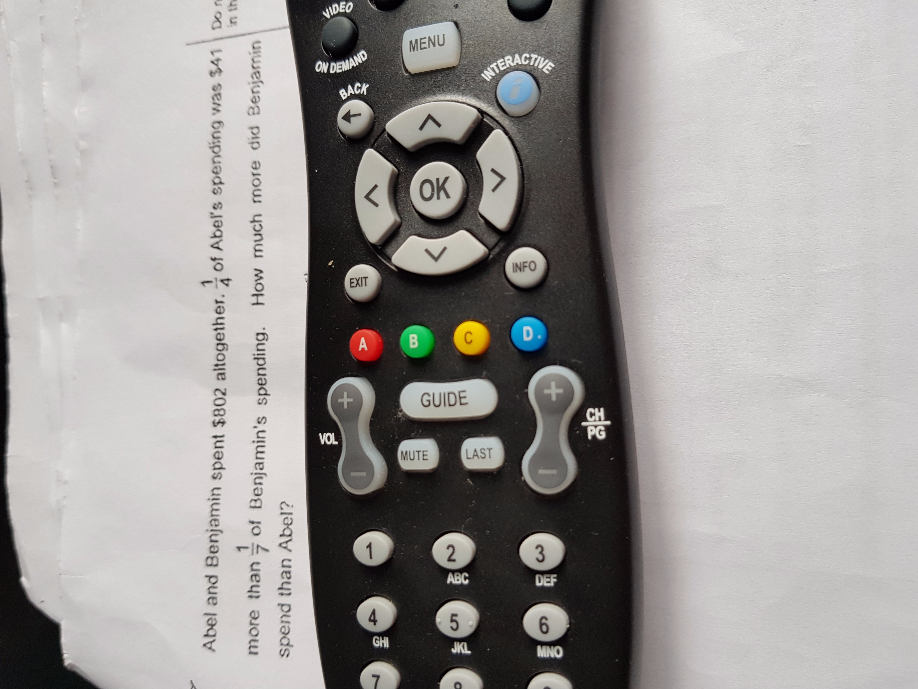QuestionHello, any one can help with this question? Thanks

Abel ——- (1u + 41) + (1u + 41) + (1u + 41) + (1u + 41) = 4u + 164
Benjamin ——- 1u + 1u + 1u + 1u + 1u + 1u + 1u = 7u

4u + 164 + 7u = 11u + 164 ——- 802
1u ——- (802 – 164)/11 = 638/11 = 58
7u – 4u – 164 = 3u – 164 ——- 3 x 58 – 164 = 10

Ans : \$10 more.
0 Replies 0 Likes

Method 1:

Benjamin spends 7u. 1/7 is 1u.

1/4 of Abel is 1u +41

4/4 of Abel is 4u + 4×41

Total spending=7u + 4u + 4×41 = 802

u=58

Benjamin spends more than Abel by

7u – 4u – 4×41 = \$10#

Method 2:

4A + 7B = 802

1A – 1B = 41 —-> 7A – 7B = 287

sum: 11A = 1089

A = 99, 4A = \$396

B = 99-41 = 58, 7B = \$406

Benjamin spends \$10 more#

0 Replies 0 Likes